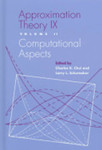## Chemistry and Physics Faculty Books and Book Chapters#### Chapter Title

Approximation to Deformations of Surfaces and its Applications

#### Book Title

Approximation Theory IX: Volume II: Computational Aspects

Book Chapter

#### Editors

Charles K. Chui, Larry L. Schumaker

#### Description

Book Abstract: This meticulously edited selection of papers comes out of the Ninth International Symposium on Approximation Theory held in Nashville, Tennessee, in January, 1998. Each volume contains several invited survey papers written by experts in the field, along with contributed research papers. This book should be of great interest to mathematicians, engineers, and computer scientists working in approximation theory, wavelets, computer-aided geometric design (CAGD), and numerical analysis. Among the topics included in the books are the following: adaptive approximation approximation by harmonic functions approximation by radial basis functions approximation by ridge functions approximation in the complex plane Bernstein polynomials bivariate splines constructions of multiresolution analyses convex approximation frames and frame bases Fourier methods generalized moduli of smoothness interpolation and approximation by splines on triangulations multiwavelet bases neural networks nonlinear approximation quadrature and cubature rational approximation refinable functions subdivision schemes thin plate splines wavelets and wavelet systems

#### ISBN

0826513271, 9780826513274

1998

#### Publisher

Vanderbilt University Press

#### Disciplines

Algebra | Analysis | Mathematics | Number TheoryCOinS